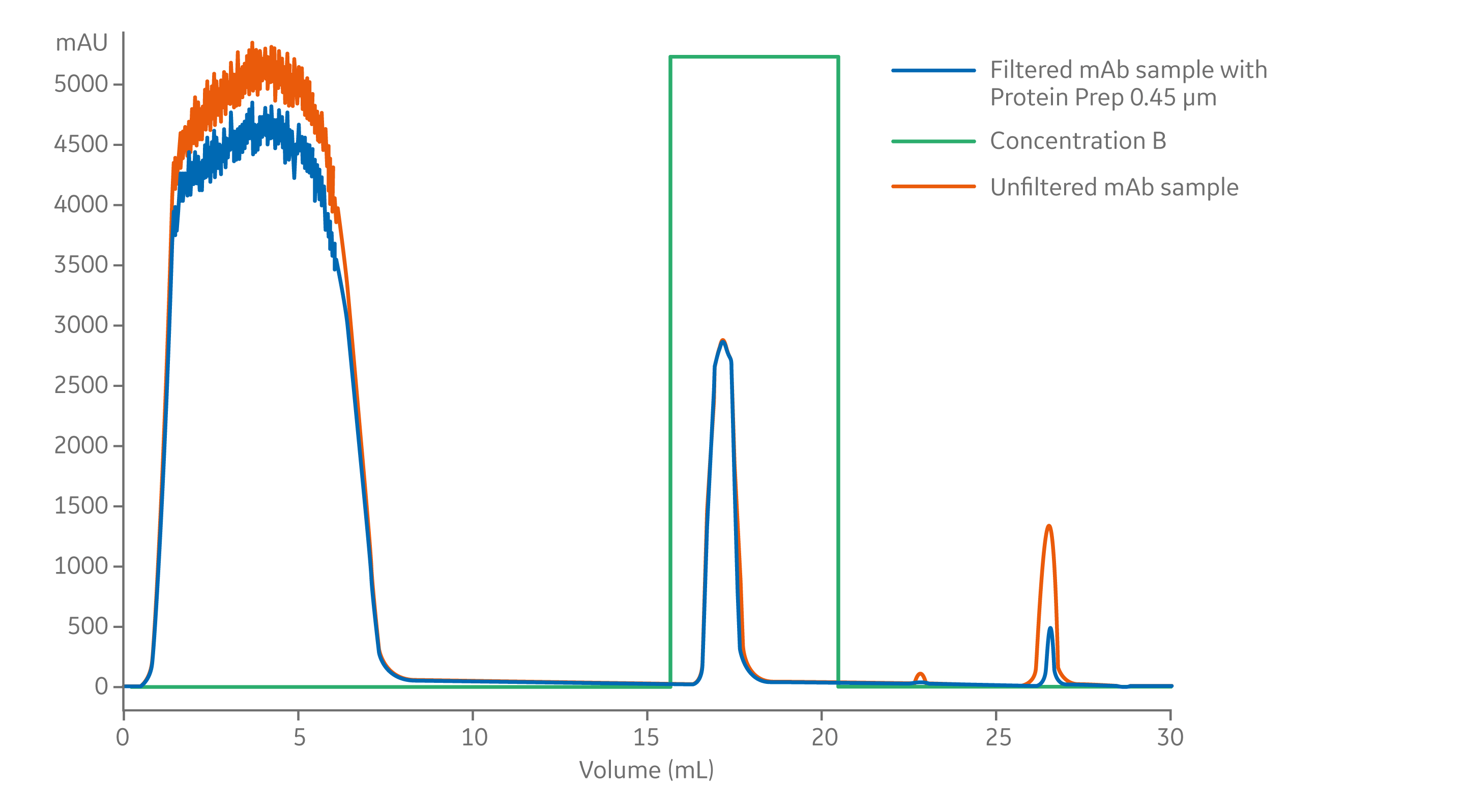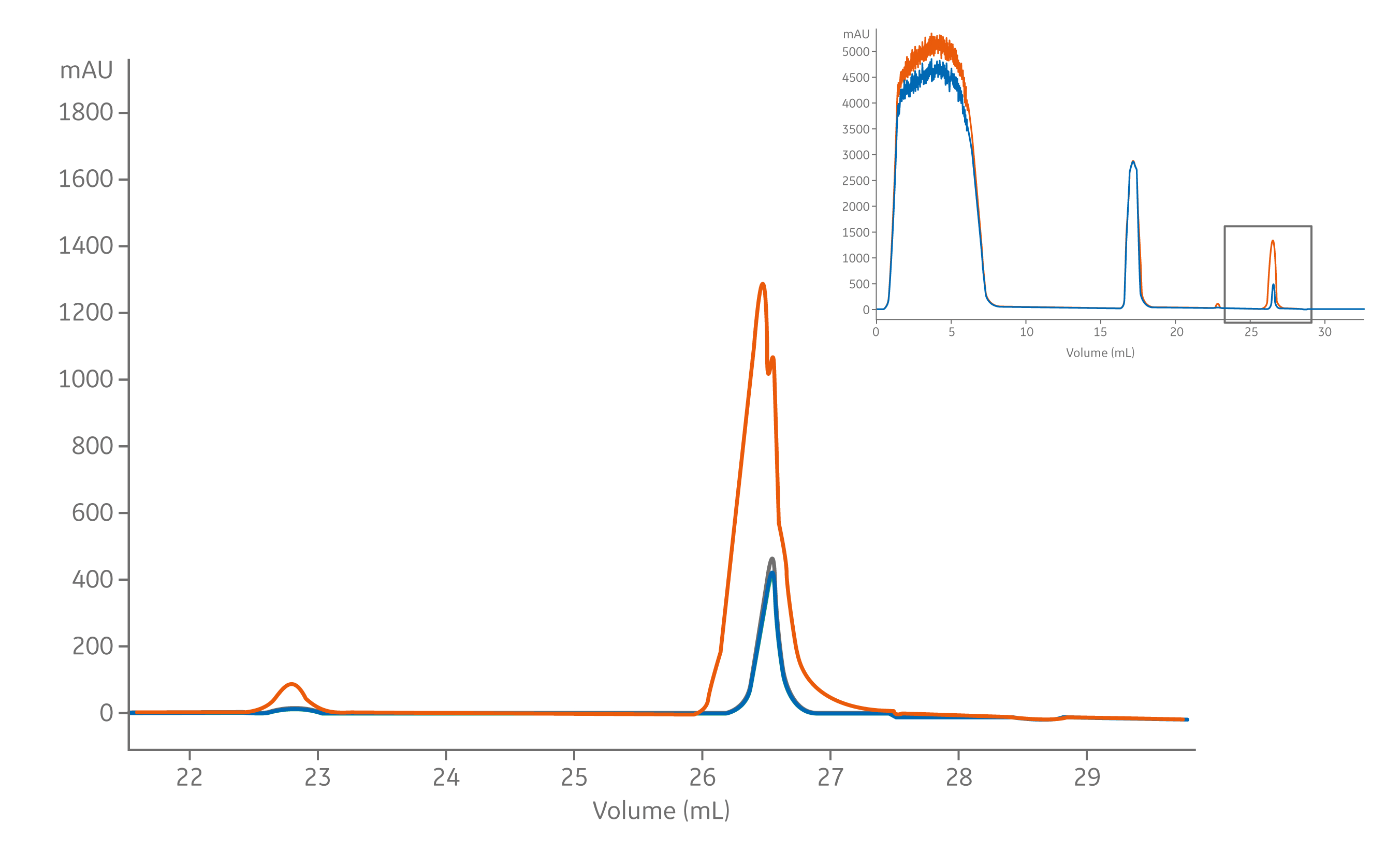孔径 1 mg/ mL (%)回收率 SD 0.5 mg/ mL (%)回收率 SD 0.2 µm 98 0.6 97 0.3 0.45 µm 99 0.7 99 0.9 SD = 标准差, N=3

 孔径 滞留体积 (µmL) 最终滞留体积 (µmL) 孔径 SD 平均值 SD 0.2 µm 135 36 9.3 0.9 0.45 µm 135 31 9.2 1.1 SD = 标准差, N= 10

A. Protein prep for ÄKTA systems 30 mm, 0.2 µm – 水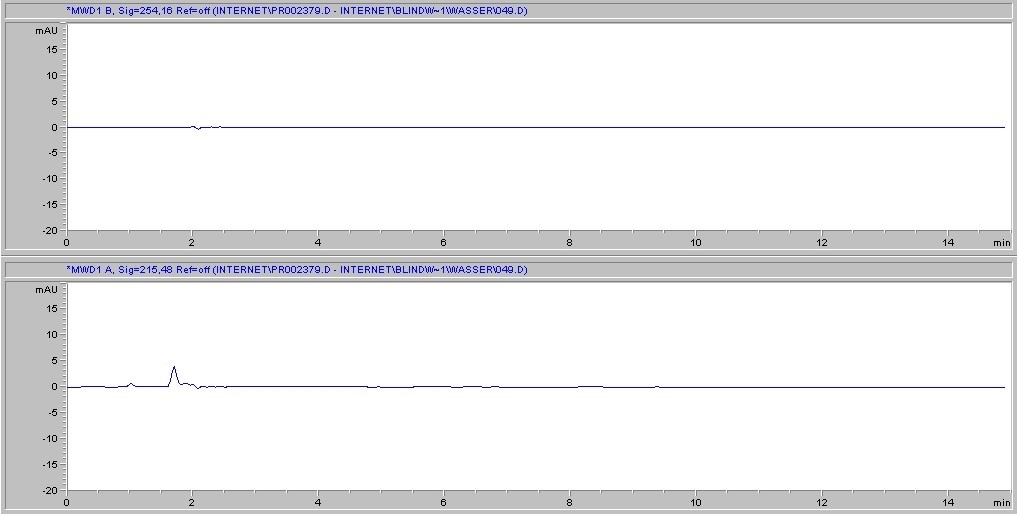B. Protein prep for ÄKTA systems 30 mm, 0.2 µm – 甲醇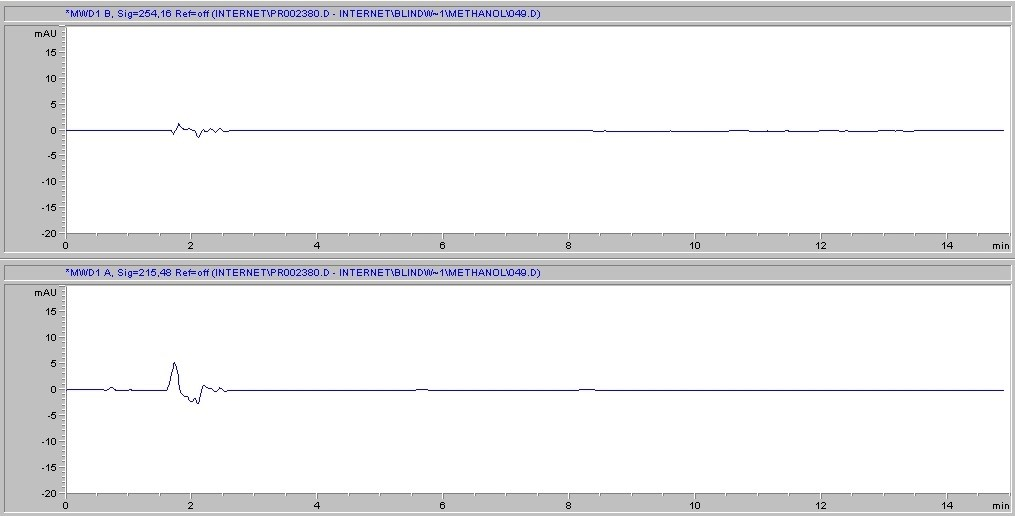C. Protein prep for ÄKTA systems 30 mm, 0.2 µm – 乙腈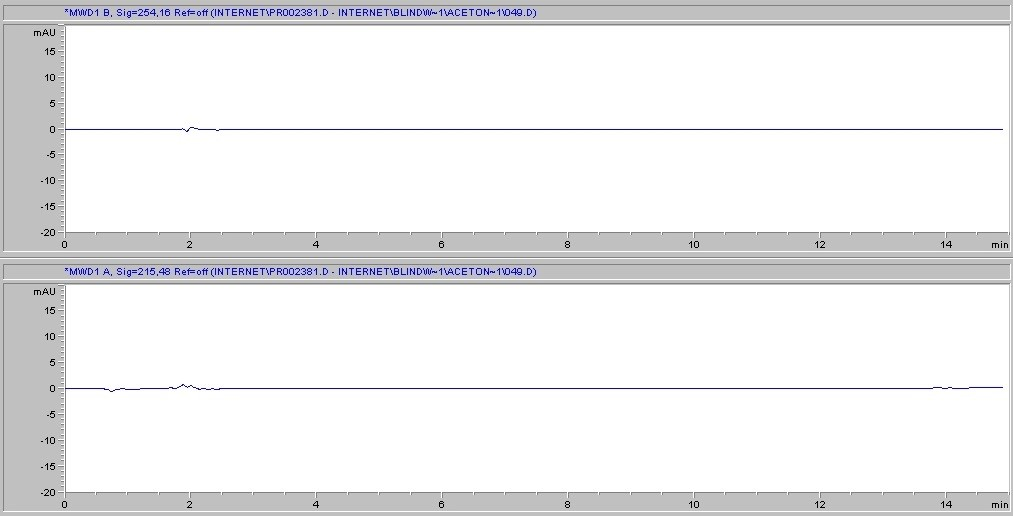溶剂 兼容性 醋酸, 5% R 冰醋酸 NR 丙酮 R 乙腈 R 氨水, 6N LR 乙酸戊酯 R 戊醇 R 苯 * R 苯甲醇 * R 硼酸 R 丁醇 R 四氯化碳 * R 三氯甲烷 * R 氯苯 * R 柠檬酸 R 甲酚 * R 环己酮 R
 溶剂 兼容性 环己烷 * R 二乙乙酰胺 R 二甲基甲酰胺 LR 二恶烷 R 二甲基亚砜 LR 乙醇 R 醚 * R 乙酸乙酯 R 乙二醇 R 甲醛 LR 蚁酸 LR 正己烷 R 浓盐酸 * NR 氢氟酸 * NR 异丁醇 R 异丙醇 R 甲醇 R
 溶剂 兼容性 丁酮 R 二氯甲烷 * R 浓硝酸 * NR 硝酸, 6N* LR 硝基苯 * R 戊烷 * R 乙烯 * R 苯酚 0.5% R 吡啶 R 氢氧化钠, 6N NR 浓硫酸, conc* NR 四氢呋喃 * R 甲苯 * R 三氯乙烷 * R 三氯乙烷 * R 水 R 二甲苯 * R
R = 耐受
LR = 有限耐受
NR = 不建议